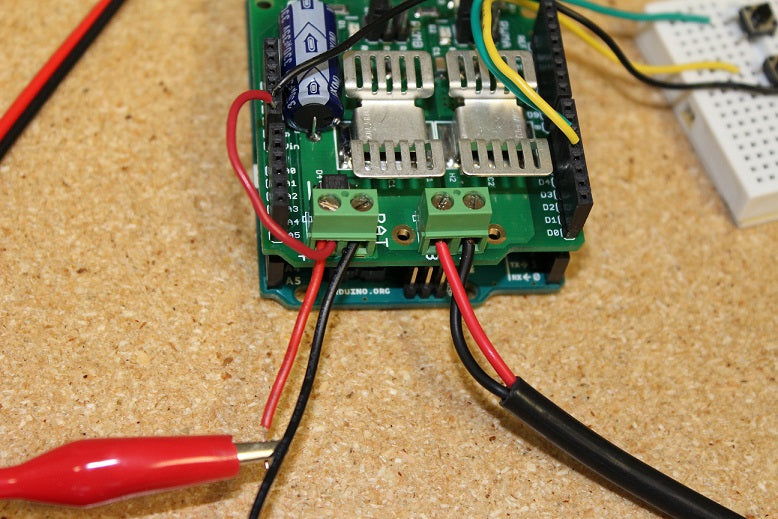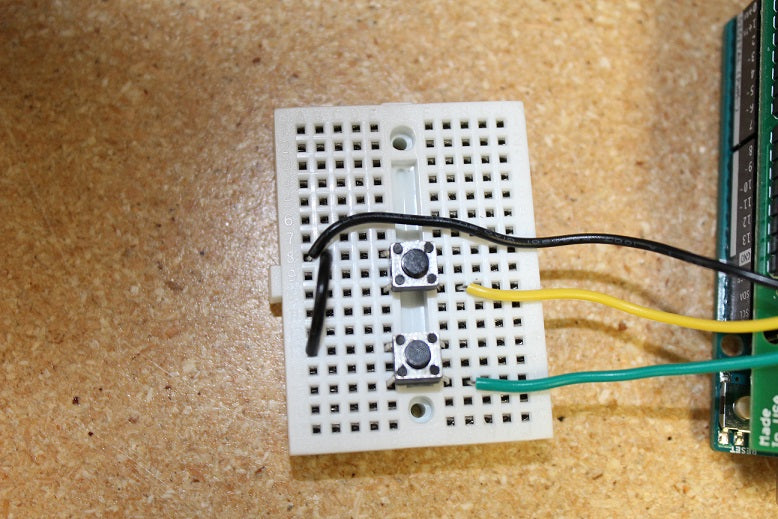# Part I: How To Monitor Feedback Of A Linear Actuator?Anna Sapiga
PA Engineer

In today’s guide, we will go over how to find out how much force a linear actuator is applying by monitoring the amount of current it is using. This will be one of our more advanced guides and will require some complex coding, calibrating and programming. We will be covering the monitoring of analog input and how it utilizes its functions. For this project, we’ll be using a MegaMoto Plus, a linear actuator (we are using our PA-14 mini actuator), an Arduino Uno and a power supply of at least 12V.To start off we’ll need to do wiring to connect everything together. Start by plugging the MegaMoto into the Arduino, just place the MegaMoto on top of the Uno to do so. Then connect a wire from the BAT+ terminal found on the MegaMoto to the Vin pin found on the Uno.Now we need to connect the linear actuators wires to the A and B terminals on the MegaMoto and connect the 12V power source to BAT+ and GND to BAT-. We’ll also need to wire two buttons for control by wiring them each between an unused pin and GND. We recommend wiring the buttons on to a breadboard.Now it is time for some coding with the Arduino Uno. We want to program the buttons so that they can control when the actuator will extend and retract. The current will start to be monitored once the actuator extends and this will let us observe whether it surpasses the max current limit or not. If it does pass the limit then the actuator will automatically stop until you choose to retract it. Taking into account that the motors inside actuators have a large current spike when they are first powered, the code we enter will have a short delay before it starts monitoring the current. This code will be able to read when the actuator has reached its limit switches, which is when the current drops to 0.
```const int EnablePin = 8;const int PWMPinA = 11;const int PWMPinB = 3; // pins for Megamotoconst int buttonLeft = 4;const int buttonRight = 5;//buttons to move the motorconst int CPin1 = A5;  // motor feedbackint leftlatch = LOW;int rightlatch = LOW;//motor latches (used for code logic)int hitLimits = 0;//start at 0int hitLimitsmax = 10;//values to know if travel limits were reached
longlastfeedbacktime = 0; // must be long, else it overflowsint firstfeedbacktimedelay = 750; //first delay to ignore current spikint feedbacktimedelay = 50; //delay between feedback cycles, how often you want the motor to be checkedcurrentTimefeedback = 0; // must be long, else it overflows
unceTime = 300; //amount to debounce buttons, lower values makes the buttons more sensitivelong lastButtonpress = 0; // timer for debouncinglong currentTimedebounce = 0;int CRaw = 0;      // input value for current readingsint maxAmps = 0; // trip limit bool dontExtend = false;bool firstRun = true;bool fullyRetracted = false;//program logic
void setup(){  Serial.begin(9600);  pinMode(EnablePin, OUTPUT);  pinMode(PWMPinA, OUTPUT);  pinMode(PWMPinB, OUTPUT);//Set motor outputs  pinMode(buttonLeft, INPUT);  pinMode(buttonRight, INPUT);//buttons   digitalWrite(buttonLeft, HIGH);  digitalWrite(buttonRight, HIGH);//enable internal pullups  pinMode(CPin1, INPUT);//set feedback input  currentTimedebounce = millis();  currentTimefeedback = 0;//Set initial times  maxAmps = 15;// SET MAX CURRENT HERE}//end setup
void loop(){  latchButtons();//check buttons, see if we need to move  moveMotor();//check latches, move motor in or out}//end main loop
void latchButtons(){  if (digitalRead(buttonLeft)==LOW)//left is forwards {    currentTimedebounce = millis() - lastButtonpress;// check time since last press    if (currentTimedebounce > debounceTime && dontExtend == false)//once you've tripped dontExtend, ignore all forwards presses    {      leftlatch = !leftlatch;// if motor is moving, stop, if stopped, start movingfirstRun = true;// set firstRun flag to ignore current spike      fullyRetracted = false; // once you move forwards, you are not fully retracted      lastButtonpress = millis();//store time of last button press      return;    }//end if  }//end btnLEFT
if (digitalRead(buttonRight)==LOW)//right is backwards  {    currentTimedebounce = millis() - lastButtonpress;// check time since last press    if (currentTimedebounce > debounceTime)    {      rightlatch = !rightlatch;// if motor is moving, stop, if stopped, start moving      firstRun = true;// set firstRun flag to ignore current spike      lastButtonpress = millis();//store time of last button press      return;        }//end if  }//end btnRIGHT}//end latchButtons
void moveMotor(){  if (leftlatch == HIGH) motorForward(255); //speed = 0-255  if (leftlatch == LOW) motorStop();  if (rightlatch == HIGH) motorBack(255); //speed = 0-255  if (rightlatch == LOW) motorStop();}//end moveMotor
void motorForward(int speeed){  while (dontExtend == false && leftlatch == HIGH)  {    digitalWrite(EnablePin, HIGH);    analogWrite(PWMPinA, speeed);    analogWrite(PWMPinB, 0);//move motor    if (firstRun == true) delay(firstfeedbacktimedelay); // bigger delay to ignore current spike    else delay(feedbacktimedelay); //small delay to get to speed    getFeedback();    firstRun = false;    latchButtons();//check buttons again  }//end while}//end motorForwardvoid motorBack (int speeed){  while (rightlatch == HIGH)  {    digitalWrite(EnablePin, HIGH);    analogWrite(PWMPinA, 0);    analogWrite(PWMPinB, speeed);//move motor    if (firstRun == true) delay(firstfeedbacktimedelay);// bigger delay to ignore current spike    else delay(feedbacktimedelay); //small delay to get to speed    getFeedback();    firstRun = false;    latchButtons();//check buttons again  }//end while  dontExtend = false;//allow motor to extend again, after it has been retracted}//end motorBack
void motorStop(){  analogWrite(PWMPinA, 0);  analogWrite(PWMPinB, 0);  digitalWrite(EnablePin, LOW);  firstRun = true;//once the motor has stopped, reenable firstRun to account for startup current spikes}//end stopMotorvoid getFeedback(){  CRaw = analogRead(CPin1); // Read current  if (CRaw == 0 && hitLimits < hitLimitsmax) hitLimits = hitLimits + 1;  else hitLimits = 0; // check to see if the motor is at the limits and the current has stopped   if (hitLimits == hitLimitsmax && rightlatch == HIGH)  {    rightlatch = LOW; // stop motor    fullyRetracted = true;  }//end if  else if (hitLimits == hitLimitsmax && leftlatch == HIGH)  {    leftlatch = LOW;//stop motor    hitLimits = 0;  }//end if  if (CRaw > maxAmps)  {    dontExtend = true;    leftlatch = LOW; //stop if feedback is over maximum  }//end if  lastfeedbacktime = millis();//store previous time for receiving feedback}//end getFeedback```